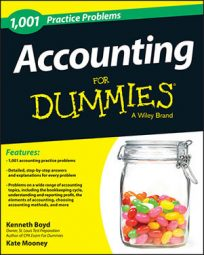##### AccountingAfter you create financial statements, you need some tools to analyze a company’s results. Following are the most frequently used formulas to analyze financial statements. Get familiar with them so that you can analyze statements with confidence.

## Components of work-in-process

Direct materials + direct labor + factory overhead applied

Work-in-process (WIP) represents cost incurred in production for partially completed goods. WIP is a subaccount within inventory. When goods are completed, they are moved to finished goods (another inventory account).

## Current ratio

Current assets ÷ current liabilities

The current ratio illustrates how easily a company can cover its current bills.

## Quick ratio

(Current assets less inventory) ÷ current liabilities

The quick ratio excludes inventory from current assets. The rationale is that inventory is the current asset that will take the longest time to convert into cash. Other current assets, such as collecting accounts receivable, may be converted into cash more quickly.

## Asset turnover ratio

Revenue (or sales) ÷ assets

This ratio explains how much profit a company generates for every dollar of assets.

## Return on equity

Net income ÷ equity

This ratio explains how much profit a company generates for every dollar of equity.

## Debt to equity ratio

Debt ÷ equity

This ratio measures what percentage of a firm’s total capitalization is debt. Capitalization refers to all funds raised by the company to operate the business.

## Contribution margin

Sales less variable costs

Contribution margin represents the amount that will be used to cover fixed costs. Any dollars remaining after paying fixed costs is considered profit.

## Return on capital

Operating profit ÷ capital

Capital is similar to equity. It represents funds raised to operate a business. Operating profit refers to profit generated from normal business activity.

## Break-even formula

Sales – variable costs – fixed costs = \$0 profit

The break-even formula calculates the level of sales that will generate a profit of \$0.

## Formula to assign overhead costs

Total overhead costs incurred ÷ activity level

Overhead costs, such as a factory’s utility costs, can’t be directly traced to a product. Instead, overhead costs are allocated based on an activity level. The activity level chosen should impact the amount of overhead costs incurred. For example, the number of machine hours used drives machinery repair costs. Machine hours should be the activity level for machine repair costs.